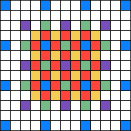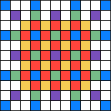# Diamond-Square — Tiled rendering and space

I started with the assumption of 128×128 pixel diamond-square tiles — they’re a power of two big (which makes them simpler to calculate), 512 bytes wide, 128 lines tall and 64KB in total size, which means two will fit in local store.

So, questions: What points outside this area are required to calculate all the points within?  How much space is required to render one of these tiles?(There’s a smaller example pictured, again with a different colour for each iteration of the calculation)

If the tile is to be 4×4, the centre area is 5×5 with overlap between tiles.  Additionally, to calculate a 4×4 tile, fully calculating a 7×7 area is required, not counting the sparsely calculated pixels around the outside.

Scaling this up to 128×128 pixel tiles and it should be clear that at least 131×131 pixel area is required, not counting those extras around the outside.  How to deal with those?

One thing that is clear is that they’re sparsely filled, and that calculated values only appear on certain lines.  In fact, the number of lines required around the outside of a tile is (1+log2n) where n is the tile width.  For the 4×4 tile pictured, three extra lines are needed on each side of the tile.  For a tile 128 lines across, 8 extra lines are needed.

So all the values to calculate a given tile can be stored in in (129+8+8)×(129+8+8) = 145×145 pixels.  Rounding up to get quadword aligned storage gives a total of 148×145×4 bytes — 85,840bytes per tile.  171,680 bytes is a big chunk of local store, but it’s probably better to be using it for something

Based on that, it’s not particularly difficult to generate the data for a tile.  Start the tile with its starting values (more on those in another post) in the corners of the squares (the blue ones in the picture), and then calculate the remaining points.  There’s some special cases required for each of the sides, but it’s nothing particularly tricky, just keeping track of row/column indexes for particular iterations.

The outer points make up only a very small percentage of the total points that need to be calculated — more than 50% of the points are calculated in the last diamond and square iterations, and due to the proximity and alignment of the points accessed, their calculation can be usefully optimised.  (I might write a post about that sometime…)